# Viz. weighted regression in SPSS and some discussionHere I wish to display some examples of visually weighted regression diagnostics in SPSS, along with some discussion about the goals and relationship to the greater visualization literature I feel is currently missing from the disscussion. To start, the current label of “visually weighted regression” can be attributed to Solomon Hsiang. Below are some of the related discussions (on both Solomon’s and Andrew Gelman’s blog), and a paper Solomon has currently posted on SSRN.

Also note that Felix Schonbrodt has provided example implementations in R. Also the last link is an example from the is.R() blog.

Hopefully that is near everyone (and I have not left out any discussion!)

A rundown of their motivation (although I encourage everyone to either read Solomon’s paper or the blog posts), is that regression estimates have a certain level of uncertainty. Particularly at the ends of the sample space of the independent variable observations, and especially for non-linear regression estimates, the uncertainty tends to be much greater than where we have more sample observations. The point of visually weighted regression is to deemphasize area of the plot where our uncertainty about the predicted values is greatest. This conversely draws ones eye to the area of the plot where the estimate is most certain.

I’ll discuss the grammar of these graphs a little bit, and from there it should be clear how to implement them in whatever software (as long as it supports transparency and fitting the necessary regression equations!). So even though I’m unaware of any current SAS (or whatever) examples, I’m sure they can be done.

## SPSS Implementation

First lets just generate some fake data in SPSS, fit a predicted regression line, and plot the intervals using lines.

``````
*******************************.
set seed = 10. /* sets random seed generator to make exact data reproducible */.
input program.
loop #j = 1 to 100. /*I typically use scratch variables (i.e. #var) when making loops.
compute X = RV.NORM(0,1). /*you can use the random number generators to make different types of data.
end case.
end loop.
end file.
end input program.
dataset name sim.
execute. /*note spacing is arbitrary and is intended to make code easier to read.
*******************************.

compute Y = 0.5*X + RV.NORM(0,SQRT(0.5)).
exe.

REGRESSION
/MISSING LISTWISE
/STATISTICS COEFF OUTS R ANOVA
/CRITERIA=PIN(.05) POUT(.10) CIN(95)
/NOORIGIN
/DEPENDENT Y
/METHOD=ENTER X
/SAVE PRED MCIN.

*Can map seperate variable based on size of interval to color and transparency.

compute size = UMCI_1 - LMCI_1.
exe.
formats UMCI_1 LMCI_1 Y X size PRE_1 (F1.0).

*Simple chart with lines.
GGRAPH
/GRAPHDATASET NAME="graphdataset" VARIABLES=X Y PRE_1 LMCI_1 UMCI_1 MISSING=LISTWISE
REPORTMISSING=NO
/GRAPHSPEC SOURCE=INLINE DEFAULTTEMPLATE=yes.
BEGIN GPL
SOURCE: s=userSource(id("graphdataset"))
DATA: X=col(source(s), name("X"))
DATA: Y=col(source(s), name("Y"))
DATA: PRE_1=col(source(s), name("PRE_1"))
DATA: LMCI_1=col(source(s), name("LMCI_1"))
DATA: UMCI_1=col(source(s), name("UMCI_1"))
GUIDE: axis(dim(1), label("X"))
GUIDE: axis(dim(2), label("Y"))
SCALE: linear(dim(1), min(-3.8), max(3.8))
SCALE: linear(dim(2), min(-3), max(3))
ELEMENT: point(position(X*Y), size(size."2"))
ELEMENT: line(position(X*PRE_1), color.interior(color.red))
ELEMENT: line(position(X*LMCI_1), color.interior(color.grey))
ELEMENT: line(position(X*UMCI_1), color.interior(color.grey))
END GPL.
``````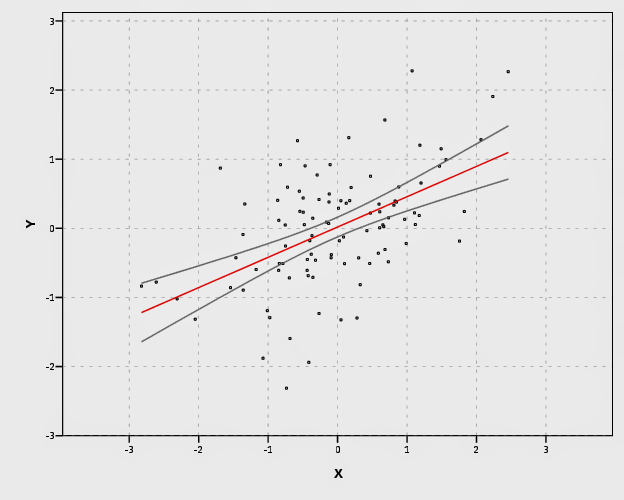In SPSS’s grammar, it is simple to plot the predicted regression line with areas of the higher confidence interval as more transparent. Here I use saved values from the regression equation, but you can also use functions within GPL (see `smooth.linear` and `region.confi.smooth`).

``````
*Using path with transparency between segments.
GGRAPH
/GRAPHDATASET NAME="graphdataset" VARIABLES=X Y PRE_1 size
/GRAPHSPEC SOURCE=INLINE.
BEGIN GPL
SOURCE: s=userSource(id("graphdataset"))
DATA: X=col(source(s), name("X"))
DATA: Y=col(source(s), name("Y"))
DATA: PRE_1=col(source(s), name("PRE_1"))
DATA: size=col(source(s), name("size"))
GUIDE: axis(dim(1), label("X"))
GUIDE: axis(dim(2), label("Y"))
GUIDE: legend(aesthetic(aesthetic.transparency.interior), null())
SCALE: linear(dim(1), min(-3.8), max(3.8))
SCALE: linear(dim(2), min(-3), max(3))
ELEMENT: point(position(X*Y), size(size."2"))
ELEMENT: line(position(X*PRE_1), color.interior(color.red), transparency.interior(size), size(size."4"))
END GPL.
``````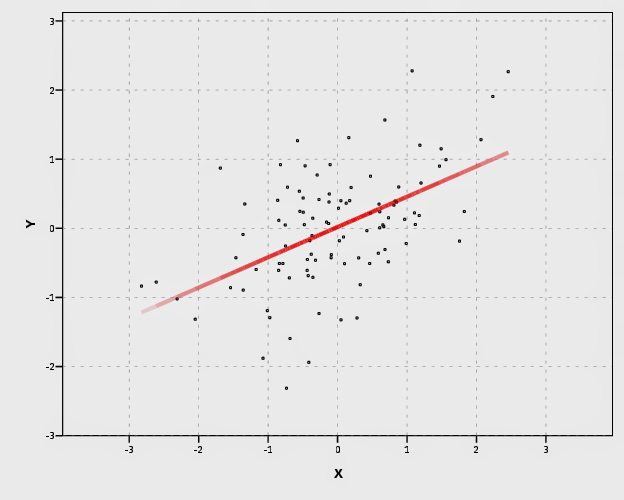It is a bit harder to make the areas semi-transparent throughout the plot. If you use an `area.difference` ELEMENT, it makes the entire area a certain amount of transparency. If you use intervals with the current data, the area will be too sparse. So what I do is make new data, sampling more densley along the x axis and make the predictions. From this we can use interval element to plot the predictions, mapping the size of the interval to transparency and color saturation.

``````
*make new cases to have a consistent sampling of x values to make the intervals.

input program.
loop #j = 1 to 500.
compute #min = -5.
compute #max = 5.
compute X = #min + (#j - 1)*(#max - #min)/500.
compute new = 1.
end case.
end loop.
end file.
end input program.
dataset name newcases.
execute.

dataset activate sim.
/file = 'newcases'.
exe.
dataset close newcases.

match files file = *
/drop UMCI_1 LMCI_1 PRE_1.

REGRESSION
/MISSING LISTWISE
/STATISTICS COEFF OUTS R ANOVA
/CRITERIA=PIN(.05) POUT(.10) CIN(95)
/NOORIGIN
/DEPENDENT Y
/METHOD=ENTER X
/SAVE PRED MCIN.

compute size = UMCI_1 - LMCI_1.
formats ALL (F1.0).

temporary.
select if new = 1.
GGRAPH
/GRAPHDATASET NAME="graphdataset" VARIABLES=X PRE_1 LMCI_1 UMCI_1 size MISSING=LISTWISE
REPORTMISSING=NO
/GRAPHSPEC SOURCE=INLINE.
BEGIN GPL
SOURCE: s=userSource(id("graphdataset"))
DATA: X=col(source(s), name("X"))
DATA: PRE_1=col(source(s), name("PRE_1"))
DATA: LMCI_1=col(source(s), name("LMCI_1"))
DATA: UMCI_1=col(source(s), name("UMCI_1"))
DATA: size=col(source(s), name("size"))
TRANS: sizen = eval(size*-1)
TRANS: sizer = eval(size*.01)
GUIDE: axis(dim(1), label("X"))
GUIDE: axis(dim(2), label("Y"))
GUIDE: legend(aesthetic(aesthetic.transparency.interior), null())
GUIDE: legend(aesthetic(aesthetic.transparency.exterior), null())
GUIDE: legend(aesthetic(aesthetic.color.saturation.exterior), null())
SCALE: linear(aesthetic(aesthetic.transparency), aestheticMinimum(transparency."0.90"), aestheticMaximum(transparency."1"))
SCALE: linear(aesthetic(aesthetic.color.saturation), aestheticMinimum(color.saturation."0"),
aestheticMaximum(color.saturation."0.01"))
SCALE: linear(dim(1), min(-5.5), max(5))
SCALE: linear(dim(2), min(-3), max(3))
ELEMENT: interval(position(region.spread.range(X*(LMCI_1 + UMCI_1))), transparency.exterior(size), color.exterior(color.red), size(size."0.005"),
color.saturation.exterior(sizen))
ELEMENT: line(position(X*PRE_1), color.interior(color.darkred), transparency.interior(sizer), size(size."5"))
END GPL.
exe.
``````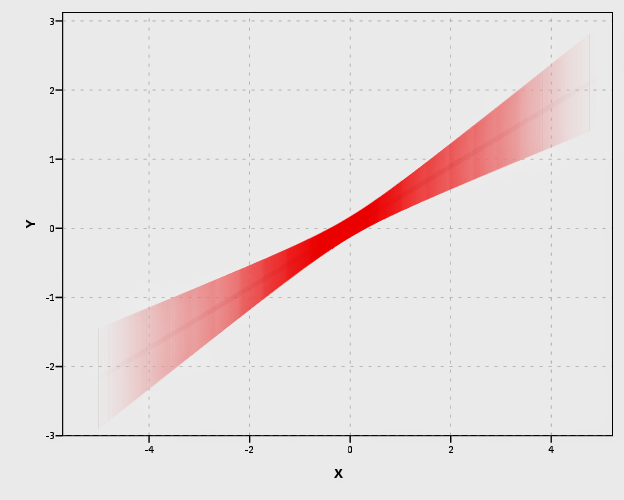Unfortunately, this creates some banding effects. Sampling fewer points makes the banding effects worse, and sampling more reduces the ability to make the plot transparent (and it is still produces banding effects). So, to produce one that looks this nice took some experimentation with how densely the new points were sampled, how aesthetics were mapped, and how wide the interval lines would be.

I tried to map a size aesthetic to the line and it works ok, you can still see some banding effects. Also note, to get the size of the line to be vertical (as oppossed to oriented in whatever direction the line is orientated) one needs to use a step line function. The `jump()` specification makes it so the vertical lines in the step chart aren’t visible. It took some trial and error to map the size to the exact interval (not sure if one can use the percent specifications to fix that trial and error). The syntax for lines though generalizes to multiple groups in SPSS easier than does the interval elements (although Solomon comments on one of Felix’s blog post that he prefers the non-interval plots with multiple groups, due to drawing difficulties and getting too busy I believe). Also FWIW it took less fussing with the density of the sample points and drawing of transparency to make the line mapped to size look nice.

``````
temporary.
select if new = 1.
GGRAPH
/GRAPHDATASET NAME="graphdataset" VARIABLES=X PRE_1 LMCI_1 UMCI_1 size MISSING=LISTWISE
REPORTMISSING=NO
/GRAPHSPEC SOURCE=INLINE.
BEGIN GPL
SOURCE: s=userSource(id("graphdataset"))
DATA: X=col(source(s), name("X"))
DATA: PRE_1=col(source(s), name("PRE_1"))
DATA: LMCI_1=col(source(s), name("LMCI_1"))
DATA: UMCI_1=col(source(s), name("UMCI_1"))
DATA: size=col(source(s), name("size"))
GUIDE: axis(dim(1), label("X"))
GUIDE: axis(dim(2), label("Y"))
GUIDE: legend(aesthetic(aesthetic.transparency.interior), null())
GUIDE: legend(aesthetic(aesthetic.transparency.exterior), null())
GUIDE: legend(aesthetic(aesthetic.size), null())
SCALE: linear(aesthetic(aesthetic.size), aestheticMinimum(size."0.16in"), aestheticMaximum(size."1.05in"))
SCALE: linear(aesthetic(aesthetic.transparency.interior), aestheticMinimum(transparency."0.5"), aestheticMaximum(transparency."1"))
SCALE: linear(dim(1), min(-5.5), max(5))
SCALE: linear(dim(2), min(-3), max(3))
ELEMENT: line(position(smooth.step.left(X*PRE_1)), jump(), color.interior(color.black), transparency.interior(size), size(size))
ELEMENT: line(position(X*PRE_1), color.interior(color.black), transparency.interior(size), size(size."3"))
END GPL.
exe.
*Add these two lines to see the actual intervals line up (without ending period).
*ELEMENT: line(position(X*LMCI_1), color.interior(color.black)).
*ELEMENT: line(position(X*UMCI_1), color.interior(color.black)).
``````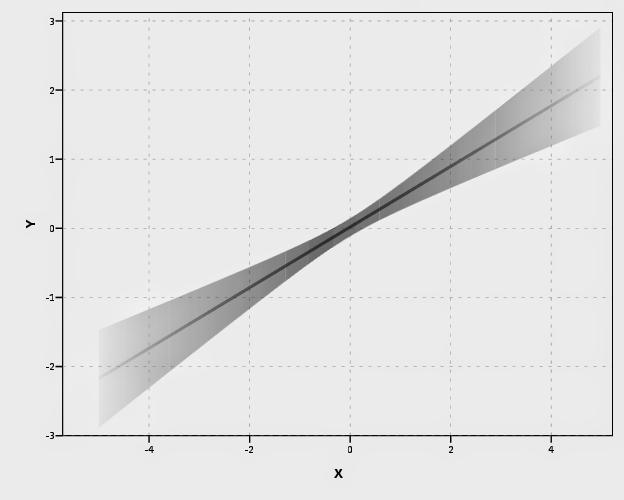Also note Solomon suggests that the saturation of the plot should be higher towards the mean of the predicted value. I don’t do that here (but have a superimposed predicted line you can see). A way to do that in SPSS would be to make multiple intervals and continually superimpose them (see Maltz and Zawitz (1998) for an applied example of something very similar). Another way may involve using color gradients for the intervals (which isn’t possible through syntax, but is through a chart template). There are other examples that I could do in SPSS (spaghetti plots of bootstrapped estimates, discrete bins for certain intervals) but I don’t provide examples of them for reasons noted below.

I will try to provide some examples of more interesting non-linear graphs in the near future (I recently made a macro to estimate restricted cubic spline bases). But this should be enough for now to show how to implement such plots in SPSS for everyone, and the necessary grammar to make the charts.

## Some notes on current discussion and implementations

My main critiques of the current implementations (and really more so the discussion) are more curmudgeonly than substantive, but hopefully it is clear enough to provide some more perspective on the work. I’ll start by saying that, it is unclear if either Solomon or Felix have read the greater visualization literature on visualizing uncertainty. While I can’t fault Felix for that so much (it is a bit much to expect a blog post to have a detailed bibliography) Solomon has at least posted a paper on SSRN. I don’t mean this as too much of a critique to Solomon, he has a good idea, and a good perspective on visualization (if you read his blog he has plenty of nice examples). But, it is more aimed at a particular set of potential alternative plots that Solomon and Felix have presented that I don’t feel are very good ideas.

Like all scientific work, we stand on the shoulders of those who come before us. While I haven’t explicitly seen visually weighted regression plots in any prior work, there are certainly many very similar examples. The most obvious would probably be the discussion of Jackson (2008), and for applied examples of this technique to very similar regression contexts are Maltz and Zawitz (1998) and Esarey and Pierce (2012) (besides Jackson (2008), there is a variety of potential other related literature cited in the discussion to Gelman’s blog posts – but this article is blatently related). There are undoubtedly many more, likely even older than the Maltz paper, and there is a treasure trove of papers about displaying error bars on this Andrew Gelman post. Besides proper attribution, this isn’t just pedantic, we should take the lessons learned from the prior literature and apply them to our current situation. There are large literatures on visualizaing uncertainty, and it is a popular enough topic that it has its own sections in cartography and visualization textbooks (MacEachren 2004; Slocum et al. 2005; Wilkinson 2005).

In particular, there is one lesson I feel should strongly reflect on the current discussion, and that is visualizing crisp lines in graphics implies the exact opposite of uncertainty to the viewers. Spiegelhalter, Pearson, and Short (2011) have an example of this, where a graphic about a tornado warning was taken a bit more to heart than it should have, and instead of people interpreting the areas of highest uncertainty in the predicted path as just that, they interpreted it as more a deterministic. There appears to be good agreement about using alpha blending (Roth, Woodruff, and Johnson 2010), and having fuzzy lines are effective ways of displaying uncertainty (MacEachren 2004; Wood et al. 2012). Thus, we have good evidence that, if the goal of the plot is to deemphasize portions of the regression that have large amounts of uncertainty in the estimates, we should not plot those estimates using discrete cut-offs. This is why I find Felix’s poll results unfortunate, in that the plot with the discrete cut-offs is voted the highest by viewers of the blog post!

So here is an example graph by Felix of the discrete bins (note this is the highest voted image in Felix’s poll!). Again to be clear, discrete bins suggests the exact opposite of uncertainty, and certainly does not deemphasize areas of the plot that have the greatest amount of uncertainty.Here is the example of the plot from Solomon that also has a similar problem with discrete bins. The colors portray uncertainty, but it is plain to see the lattice on the plot, and I don’t understand why this meets any of Solomon’s original goals. It takes ink to plot the lattice!More minor, but still enough to guide our implementations, the plots that superimpose multiple bootstrapped estimates, while they are likely ok to visualize uncertainty, the resulting spaghetti make the plots much more complex. The shaded areas maintain a much more condense and clear to understand visual, while superimposing multiple lines on the plot creates a difficult to envision distribution (here I have an example on the CV blog, and it is taken from Carr and Pickle (2009)). It may aid understanding uncertainty about the regression estimate, but it detracts from visualizing any more global trends in the regression estimate. It also fails to meet the intial goal of deemphasizing areas of the plot that are most uncertain. It accomplishes quite the opposite actually, and areas where the bootstrapped estimates have a greater variance will draw more attention because they are more dispersed on the plot.

Here is an example from Solomon’s blog of the spaghetti plots with the lines drawn heavily transparent.Solomon writes about the spaghetti plot distraction in his SSRN paper, but still presents the examples as if they are reasonable alternatives (which is strange). I would note these would be fine and dandy if visualizing the uncertainty was itself an original goal of the plot, but that isn’t the goal! To a certain extent, displaying an actual interval is countervailing to the notion of deemphasizing that area of the chart. The interval needs to command a greater area of the chart. I think Solomon has some very nice examples where the tradeoff is reasonable, with plotting the areas with larger intervals in lower color saturation (here I use transparency to the same effect). I doubt this is news to Solomon – what he writes in his SSRN paper conforms to what I’m saying as far as I can tell – I’m just confused why he presents some examples as if they are reasonable alternatives. I think it deserves reemphasis though given all the banter and implementations floating around the internet, especially with some of the alternatives Felix has presented.

I’m not sure if Solomon and Felix really appreciate though the distinction between hard lines and soft lines though after reading the blog post(s) and the SSRN paper. Of course these assertions and critiques of mine should be tested in experimental settings, but we should not ignore prior research in spite of a lack of experimental findings. I don’t want these critiques to be viewed too harshly though, and I hope Solomon and Felix take them to heart (either in future implementations or actual papers discussing the technique).

Carr, Daniel B., and Linda Williams Pickle. 2009. Visualizing Data Patterns with Micromaps. Boca Rotan, FL: CRC Press.

Esarey, Justin, and Andrew Pierce. 2012. Assessing fit quality and testing for misspecification in binary-dependent variable models. Political Analysis 20: 480–500.

Hsiang, Solomon M. 2012. Visually-Weighted Regression.

Jackson, Christopher H. 2008. Displaying uncertainty with shading. The American Statistician 62: 340–47.

MacEachren, Alan M. 2004. How maps work: Representation, visualization, and design. New York, NY: The Guilford Press.

Maltz, Michael D., and Marianne W. Zawitz. 1998. Displaying violent crime trends using estimates from the National Crime Victimization Survey. US Department of Justice, Office of Justice Programs, Bureau of Justice Statistics.

Roth, Robert E., Andrew W. Woodruff, and Zachary F. Johnson. 2010. Value-by-alpha maps: An alternative technique to the cartogram. The Cartographic Journal 47: 130–40.

Slocum, Terry A., Robert B. McMaster, Fritz C. Kessler, and Hugh H. Howard. 2005. Thematic cartography and geographic visualization. Prentice Hall.

Spiegelhalter, David, Mike Pearson, and Ian Short. 2011. Visualizing uncertainty about the future. Science 333: 1393–1400.

Wilkinson, Leland. 2005. The grammar of graphics. New York, NY: Springer.

Wood, Jo, Petra Isenberg, Tobias Isenberg, Jason Dykes, Nadia Boukhelifa, and Aidan Slingsby. 2012. Sketchy Rendering for Information Visualization. Visualization and Computer Graphics, IEEE Transactions on 18: 2749–58.

# Fluctuation diagrams in SPSS

The other day on my spineplot post Jon Peck made a comment about how he liked the structure charts in this CV thread. Here I will show how to make them in SPSS (FYI the linked thread has an example of how to make them in R using ggplot2 if you are interested).

Unfortunately I accidently called them a structure plot in the original CV thread, when they are actually called fluctuation diagrams (see Pilhofer et al. (2012) and Wickham & Hofmann (2011) for citations). They are basically just binned scatterplots for categorical data, and the size of a point is mapped to the number of observations that fall within that bin. Below is the example (in ggplot2) taken from the CV thread.So, to make these in SPSS you first need some categorical data, you can follow along with any two categorical variables (or at the end of the post I have the complete syntax with some fake categorical data). First, it is easier to start with some boilerplate code generated through the GUI. If you have any data set open that has categorical data in it (unaggregated) you can simply open up the chart builder dialog, choose a barplot, place the category you want on the x axis, then place the category you want on the Y axis as a column panel for a paneled bar chart.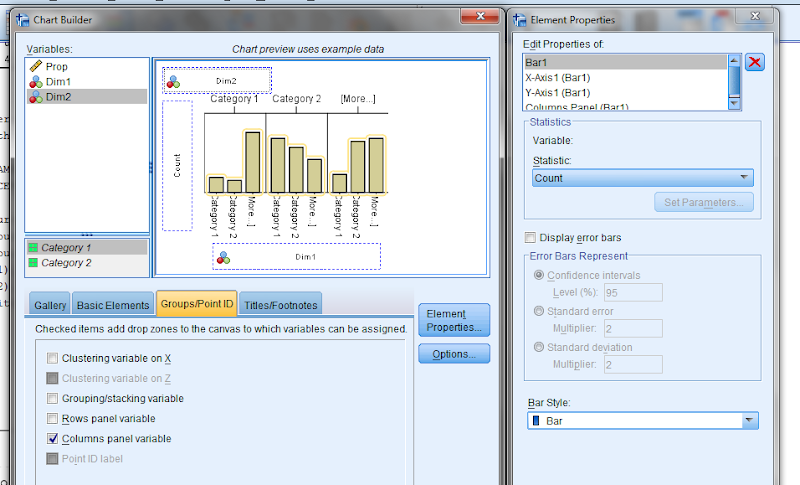The reason you make this chart is that the GUI interprets the default behavior of this bar chart is to aggregate the frequencies. You make the colum panel just so the GUI will write out the data definitions for you. If you pasted the chart builder syntax then the GPL code will look like below.

``````
*Fluctuation plots - first make column paneled bar chart.
GGRAPH
/GRAPHDATASET NAME="graphdataset" VARIABLES=Dim1[LEVEL=NOMINAL] COUNT()
[name="COUNT"] Dim2[LEVEL=NOMINAL] MISSING=LISTWISE REPORTMISSING=NO
/GRAPHSPEC SOURCE=INLINE.
BEGIN GPL
SOURCE: s=userSource(id("graphdataset"))
DATA: Dim1=col(source(s), name("Dim1"), unit.category())
DATA: COUNT=col(source(s), name("COUNT"))
DATA: Dim2=col(source(s), name("Dim2"), unit.category())
GUIDE: axis(dim(1), label("Dim1"))
GUIDE: axis(dim(2), label("Count"))
GUIDE: axis(dim(3), label("Dim2"), opposite())
SCALE: cat(dim(1))
SCALE: linear(dim(2), include(0))
SCALE: cat(dim(3))
ELEMENT: interval(position(Dim1*COUNT*Dim2), shape.interior(shape.square))
END GPL.
``````

With this boilerplate code though we can edit to make the chart we want. Here I outline some of those steps. Only editing the `ELEMENT` portion, the steps below are;

• Edit the `ELEMENT` statement to be a `point` instead of `interval`.
• Delete `COUNT` within the position statement (within the `ELEMENT`).
• Change `shape.interior` to `shape`.
• Add in `,size(COUNT)` after `shape(shape.square)`.
• Add in `,color.interior(COUNT)` after `size(COUNT)`.

Those are all of the necessary statements to produce the fluctuation chart. The next two are to make the chart look nicer though.

• Add in aesthetic mappings for scale statements (both the color and the size).
• Change the guide statements to have the correct labels (and delete the dim(3) GUIDE).

The GPL code call then looks like this (with example aesthetic mappings) and below that is the chart it produces.

``````
*In the end fluctuation plot.
GGRAPH
/GRAPHDATASET NAME="graphdataset" VARIABLES=Dim1 Dim2 COUNT()[name="COUNT"]
/GRAPHSPEC SOURCE=INLINE.
BEGIN GPL
SOURCE: s=userSource(id("graphdataset"))
DATA: Dim1=col(source(s), name("Dim1"), unit.category())
DATA: Dim2=col(source(s), name("Dim2"), unit.category())
DATA: COUNT=col(source(s), name("COUNT"))
GUIDE: axis(dim(1), label("Dim1"))
GUIDE: axis(dim(2), label("Dim2"))
SCALE: pow(aesthetic(aesthetic.size), aestheticMinimum(size."8px"), aestheticMaximum(size."45px"))
SCALE: linear(aesthetic(aesthetic.color.interior), aestheticMinimum(color.lightgrey), aestheticMaximum(color.darkred))
ELEMENT: point(position(Dim1*Dim2), shape(shape.square), color.interior(COUNT), size(COUNT))
END GPL.
``````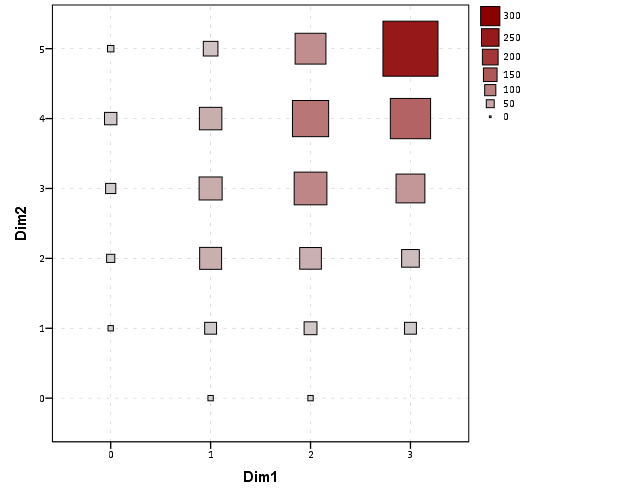Aesthetically, besides the usual niceties the only thing to note is that the size of the squares typically needs to be changed to fill up the space (you would have to be lucky to have an exact mapping between area and the categorical count to work out). I presume squares are preferred because area assessments with squares tend to be more accurate than circles, but that is just my guess (you could use any shape you wanted). I use a power scale for size aesthetic, as the area for a square increases by the size of the side squared (and people interpret the areas in the plot, not the size of the side of the square). SPSS’s default exponent for a power scale is 0.5, which is the square root so exactly what we want. You just need to supply a reasonable start and end size for the squares to let them fill up the space depending on your counts. Unfortunately, SPSS does not make a correctly scaled legend in size, but the color aesthetic is correct (I leave it in only to show that it is incorrect, if for publication I would like suppress the different sizes and only show the color gradient). (Actually, my V20 continues to not respect shape aesthetics that aren’t mapped – and this is produced via post-hoc editing of the shape – owell).

Here I show two redundant continuous aesthetic scales (size and color). SPSS’s behavior is to make the legend discrete instead of continuous. In Wilkinson’s Grammar of Graphics he states that he prefers discrete scales (even for continous aesthetics) to aid lookup.

``````
***********************************************************.
*Making random categorical data.
set seed 14.
input program.
loop #i = 1 to 1000.
compute Prop = RV.UNIFORM(.5,1).
end case.
end loop.
end file.
end input program.
dataset name cats.
exe.

compute Dim1 = RV.BINOMIAL(3,PROP).
compute Dim2 = RV.BINOMIAL(5,PROP).

*Fluctuation plots - first make column paneled bar chart.
GGRAPH
/GRAPHDATASET NAME="graphdataset" VARIABLES=Dim1[LEVEL=NOMINAL] COUNT()
[name="COUNT"] Dim2[LEVEL=NOMINAL] MISSING=LISTWISE REPORTMISSING=NO
/GRAPHSPEC SOURCE=INLINE.
BEGIN GPL
SOURCE: s=userSource(id("graphdataset"))
DATA: Dim1=col(source(s), name("Dim1"), unit.category())
DATA: COUNT=col(source(s), name("COUNT"))
DATA: Dim2=col(source(s), name("Dim2"), unit.category())
GUIDE: axis(dim(1), label("Dim1"))
GUIDE: axis(dim(2), label("Count"))
GUIDE: axis(dim(3), label("Dim2"), opposite())
SCALE: cat(dim(1))
SCALE: linear(dim(2), include(0))
SCALE: cat(dim(3))
ELEMENT: interval(position(Dim1*COUNT*Dim2), shape.interior(shape.square))
END GPL.

*Then edit 1) element to point.
*2) delete COUNT within position statement
*3) shape.interior -> shape
*6) add in aesthetic mappings for scale statements
*7) change guide statements - then you are done.

*In the end fluctuation plot.
GGRAPH
/GRAPHDATASET NAME="graphdataset" VARIABLES=Dim1 Dim2 COUNT()[name="COUNT"]
/GRAPHSPEC SOURCE=INLINE.
BEGIN GPL
SOURCE: s=userSource(id("graphdataset"))
DATA: Dim1=col(source(s), name("Dim1"), unit.category())
DATA: Dim2=col(source(s), name("Dim2"), unit.category())
DATA: COUNT=col(source(s), name("COUNT"))
GUIDE: axis(dim(1), label("Dim1"))
GUIDE: axis(dim(2), label("Dim2"))
SCALE: pow(aesthetic(aesthetic.size), aestheticMinimum(size."8px"), aestheticMaximum(size."45px"))
SCALE: linear(aesthetic(aesthetic.color.interior), aestheticMinimum(color.lightgrey), aestheticMaximum(color.darkred))
ELEMENT: point(position(Dim1*Dim2), shape(shape.square), color.interior(COUNT), size(COUNT))
END GPL.

*Alternative ways to map sizes in the plot.
*SCALE: linear(aesthetic(aesthetic.size), aestheticMinimum(size."5%"), aestheticMaximum(size."30%")).
*SCALE: linear(aesthetic(aesthetic.size), aestheticMinimum(size."6px"), aestheticMaximum(size."18px")).

*Alternative jittered scatterplot - need to remove the agg variable COUNT.
*Replace point with point.jitter.
GGRAPH
/GRAPHDATASET NAME="graphdataset" VARIABLES=Dim1 Dim2
/GRAPHSPEC SOURCE=INLINE.
BEGIN GPL
SOURCE: s=userSource(id("graphdataset"))
DATA: Dim1=col(source(s), name("Dim1"), unit.category())
DATA: Dim2=col(source(s), name("Dim2"), unit.category())
GUIDE: axis(dim(1), label("Dim1"))
GUIDE: axis(dim(2), label("Dim2"))
ELEMENT: point.jitter(position(Dim1*Dim2))
END GPL.
***********************************************************.
``````

# The Junk Charts Challenge: Remaking a great line chart in SPSS

I read and very much enjoy Kaiser Fung’s blog Junk Charts. One of the exchanges in the comments to the post, Remaking a great chart, Kaiser asserted it was easier to make the original chart in Excel than in any current programming language. I won’t deny it is easier to use a GUI dialog than learn some code, but here I will present how you would go about making the chart in SPSS’s grammar of graphics. The logic extends part-and-parcel to ggplot2.

The short answer is the data is originally in wide format, and most statistical packages it is only possible (or at least much easier) to make the chart when the data is in long format. This ends up being not a FAQ, but a frequent answer to different questions, so I hope going over such a task will have wider utility for alot of charting tasks.

So here is the original chart (originally from the Calculated Risk blog)And here is Kaiser Fung’s updated version;Within the article Kaiser states;

One thing you’ll learn quickly from doing this exercise is that this is a task ill-suited for a computer (so-called artificial intelligence)! The human brain together with Excel can do this much faster. I’m not saying you can’t create a custom-made application just for the purpose of creating this chart. That can be done and it would run quickly once it’s done. But I find it surprising how much work it would be to use standard tools like R to do this.

Of course because anyone saavy with a statistical package would call bs (because it is), Kaiser gets some comments by more experienced R users saying so. Then Kaiser retorts in the comments with a question how to go about making the charts in R;

Hadley and Dean: I’m sure you’re better with R than most of us so I’d love to hear more. I have two separate issues with this task:

1. assuming I know exactly the chart to build, and have all the right data elements, it is still much easier to use Excel than any coding language. This is true even if I have to update the chart month after month like CR blog has to. I see this as a challenge to those creating graphing software. (PS. Here, I’m thinking about the original CR version – I don’t think that one can easily make small multiples in Excel.)
1. I don’t see a straightforward way to proceed in R (or other statistical languages) from grabbing the employment level data from the BLS website, and having the data formatted precisely for the chart I made. Perhaps one of you can give us some pseudo-code to walk through how you might do it. I think it’s easier to think about it than to actually do it.

So here I will show how one would go about making the charts in a statistical package, here SPSS. I actually don’t use the exact data to make the same chart, but there is very similar data at the Fed Bank of Minneapolis website. Here I utilize the table on cumulative decline of Non-Farm employment (seasonally adjusted) months after the NBER defined peak. I re-format the data so it can actually be read into a statistical package, and here is the xls data sheet. Also at that link the zip file contains all the SPSS code needed to reproduce the charts in this blogpost.

So first up, the data from the Fed Bank of Minneapolis website looks like approximately like this (in csv format);

``````MAP,Y1948,Y1953,Y1957,Y1960,Y1969,Y1973,Y1980,Y1981,Y1990,Y2001,Y2007
0,0,0,0,0,0,0,0,0,0,0,0
1,-0.4,-0.1,-0.4,-0.6,-0.1,0.2,0.1,0.0,-0.2,-0.2,0.0
2,-1.1,-0.3,-0.7,-0.8,0.1,0.3,0.2,-0.1,-0.3,-0.2,-0.1
3,-1.5,-0.6,-1.1,-0.9,0.3,0.4,0.1,-0.2,-0.4,-0.3,-0.1
4,-2.1,-1.2,-1.4,-1.0,0.2,0.5,-0.4,-0.5,-0.5,-0.4,-0.3``````

This isn’t my forte, so I’m unsure when Kaiser says grab the employment level data from the BLS website what exact data or table he is talking about. Regardless, if the table you grab the data from is in this wide format, it will be easier to make the charts we want if the data is in long format. So in the end if you want the data in long format, instead of every line being a different column, all the lines are in one column, like so;

``````MAP, YEAR, cdecline
0, 1948, 0
1, 1948, -.04
.
72, 1948, 8.2
0, 2007, 0
1, 2007, 0
.``````

So in SPSS, the steps would be like this to reshape the data (after reading in the data from my prepped xls file);

``````GET DATA /TYPE=XLS
/FILE='data\historical_recessions_recoveries_data_03_08_2013.xls'
/SHEET=name 'NonFarmEmploy'
/CELLRANGE=full
/ASSUMEDSTRWIDTH=32767.
DATASET NAME NonFarmEmploy.

*Reshape wide to long.
VARSTOCASES
/MAKE cdecline from Y1948 to Y2007
/INDEX year (cdecline).
compute year = REPLACE(year,"Y","").``````

This produces the data so instead of having seperate years in different variables, you have the cumulative decline in one column in the dataset, and another categorical variable identifying the year. Ok, so now we are ready to make a chart that replicates the original from the calculated risk blog. So here is the necessary code in SPSS to make a well formatted chart. Note the compute statement first makes a variable to flag if the year is 2007, which I then map to the aesthetics of red and larger size, so it comes to the foreground of the chart;

``````compute flag_2007 = (year = "2007").
GGRAPH
/GRAPHDATASET NAME="graphdataset" VARIABLES=MAP cdecline flag_2007 year
/GRAPHSPEC SOURCE=INLINE.
BEGIN GPL
SOURCE: s=userSource(id("graphdataset"))
DATA: MAP=col(source(s), name("MAP"))
DATA: cdecline=col(source(s), name("cdecline"))
DATA: flag_2007=col(source(s), name("flag_2007"), unit.category())
DATA: year=col(source(s), name("year"), unit.category())
SCALE: cat(aesthetic(aesthetic.color), map(("0", color.grey), ("1", color.red)))
SCALE: cat(aesthetic(aesthetic.size), map(("0",size."1px"), ("1",size."3.5px")))
SCALE: linear(dim(1), min(0), max(72))
SCALE: linear(dim(2), min(-8), max(18))
GUIDE: axis(dim(1), label("Months After Peak"), delta(6))
GUIDE: axis(dim(2), label("Cum. Decline from NBER Peak"), delta(2))
GUIDE: form.line(position(*,0), size(size."1px"), shape(shape.dash), color(color.black))
GUIDE: legend(aesthetic(aesthetic.color.interior), null())
GUIDE: legend(aesthetic(aesthetic.size), null())
ELEMENT: line(position(MAP*cdecline), color(flag_2007), size(flag_2007), split(year))
END GPL.``````

Which produces this chart (ok I cheated alittle, I post-hoc added the labels in by hand in the SPSS editor, as I did not like the automatic label placement and it is easier to add in by hand than fix the automated labels). Also note this will appear slightly different than the default SPSS charts because I use my own personal chart template.That is one hell of a chart command call though! You can actually produce most of the lines for this call through SPSS’s GUI dialog, and it just takes some more knowledge of the graphic language of SPSS to adjust the aesthetics of the chart. It would take a book to go through exactly how GPL works and the structure of the grammar, but here is an attempt at a more brief run down.

So typically, you would make seperate lines by specifiying that every year gets its own color. This is nearly impossible to distinguish between all of the lines though (as Kaiser originally states). A simple solution is to only highlight the line we are interested in, 2007, and make the rest of the lines the same color. To do this and still have the lines rendered seperately in SPSS’s GPL code, one need to specify the `split` modifier within the `ELEMENT` statement (the equivalent in ggplot2 is the group statement within aes). The things I manually edited differently than the original code generated through the GUI are;

• Guide line at the zero value, and then making the guideline 1 point wide, black, and with a dashed pattern (`GUIDE: form.line`)
• Color and size the 2007 line differently than the rest of the lines (`SCALE: cat(aesthetic(aesthetic.color), map(("0", color.grey), ("1", color.red)))`)
• Set the upper and lower boundary of the x and y axis (`SCALE: linear(dim(2), min(-8), max(18))`)
• set the labels for the x and y axis, and set how often tick marks are generated (`GUIDE: axis(dim(2), label("Cum. Decline from NBER Peak"), delta(2))`)
• set the chart so the legend for the mapped aesthetics are not generated, because I manually label them anyway (`GUIDE: legend(aesthetic(aesthetic.size), null())`)

Technically, both in SPSS (and ggplot2) you could produce the chart in the original wide format, but this ends up being more code in the chart call (and grows with the numbers of groups) than simply reshaping the data so the data to makes the lines is in one column.

This chart, IMO, makes the point we want to make easily and succintly. The recession in 2007 has had a much harsher drop off in employment and has lasted much longer than employment figures in any recession since 1948. All of the further small multiples are superflous unless you really want to drill down into the differences between prior years, which are small in magnitude compared to the current recession. Using small lines and semi-transparency is the best way to plot many lines (and I wish people running regressions on panel data sets did it more often!)

So although that one graph call is complicated, it takes relatively few lines of code to read in the data and make it. In ggplot2 I’m pretty sure would be fewer lines (Hadley’s version of the grammar is much less verbose than SPSS). So, in code golf terms of complexity, we are doing alright. The power in programming though is it is trivial to reuse the code. So to make a paneled version similar to Kaiser’s remake we simply need to make the panel groupings, then copy-paste and slightly update the prior code to make a new chart;

``````compute #yearn = NUMBER(year,F4.0).
if RANGE(#yearn,1940,1959) = 1 decade = 1.
if RANGE(#yearn,1960,1979) = 1 decade = 2.
if RANGE(#yearn,1980,1999) = 1 decade = 3.
if RANGE(#yearn,2000,2019) = 1 decade = 4.
1 '1940s-50s'
2 '1960s-70s'
3 '1980s-90s'
4 '2000s'.

GGRAPH
/GRAPHDATASET NAME="graphdataset" VARIABLES=MAP cdecline year decade flag_2007
/GRAPHSPEC SOURCE=INLINE.
BEGIN GPL
SOURCE: s=userSource(id("graphdataset"))
DATA: MAP=col(source(s), name("MAP"))
DATA: cdecline=col(source(s), name("cdecline"))
DATA: year=col(source(s), name("year"), unit.category())
DATA: flag_2007=col(source(s), name("flag_2007"), unit.category())
SCALE: cat(aesthetic(aesthetic.color), map(("0", color.black), ("1", color.red)))
SCALE: cat(aesthetic(aesthetic.size), map(("0",size."1px"), ("1",size."3.5px")))
SCALE: linear(dim(1), min(0), max(72))
SCALE: linear(dim(2), min(-8), max(18))
GUIDE: axis(dim(1), label("Months After Peak"), delta(6))
GUIDE: axis(dim(2), label("Cum. Decline from NBER Peak"), delta(2))
GUIDE: axis(dim(4), opposite())
GUIDE: form.line(position(*,0), size(size."0.5px"), shape(shape.dash), color(color.lightgrey))
GUIDE: legend(aesthetic(aesthetic.color), null())
GUIDE: legend(aesthetic(aesthetic.size), null())
END GPL.``````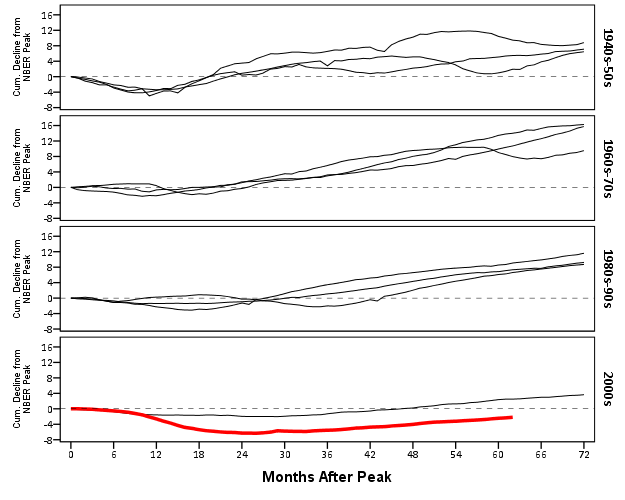It should be easy to see comparing the new paneled chart syntax to the original, it only took two slight changes; 1) I needed to add in the new decade variable and define it in the `DATA` mapping, 2) I needed to add it to the `ELEMENT` call to produce paneling by row. Again I cheated alittle, I post hoc edited the grid lines out of the image, and changed the size of the Y axis labels. If I really wanted to automate these things in SPSS, I would need to rely on a custom template. In R in ggplot2, this is not necessary, as everything is exposed in the programming language. This is quite short work. Harder is to add in labels, I don’t bother here, but I would assume to do it nicely (if really needed) I would need to do it manually. I don’t bother here because it isn’t clear to me why I should care about which prior years are which.

On aesthetics, I would note Kaiser’s original panelled chart lacks distinction between the panels, which makes it easy to confuse Y axis values. I much prefer the default behavior of SPSS here. Also the default here does not look as nice in the original in terms of the X to Y axis ratio. This is because the panels make the charts Y axis shrink (but keep the X axis the same). My first chart I suspect looks nicer because it is closer to the Cleveland ideal of average 45 degree banking in the line slopes.

What about the data manipulation Kaiser suggests is difficult to conduct in a statistical programming language? Well, that is more difficult, but certainly not impossible (and certainly not faster in Excel to anyone who knows how to do it!) Here is how I would go about it in SPSS to identify the start, the trough, and the recovery.

``````*Small multiple chart in piecewise form, figure out start, min and then recovery.
compute flag = 0.
*Start.
if MAP = 0 flag = 1.
*Min.
sort cases by year cdecline.
do if year <> lag(year) or \$casenum = 1.
compute flag = 2.
compute decline_MAP = MAP.
else if year = lag(year).
compute decline_MAP = lag(decline_MAP).
end if.
*Recovery.
*I need to know if it is after min to estimate this, some have a recovery before the
min otherwise.
sort cases by year MAP.
if lag(cdecline) < 0 and cdecline >= 0 and MAP > decline_MAP flag = 3.
if year = "2007" and MAP = 62 flag = 3.
exe.
*Now only select these cases.
dataset copy reduced.
dataset activate reduced.
select if flag > 0.``````

So another 16 lines (that aren’t comments) – what is this world of complex statistical programming coming too! If you want a run-down of how I am using lagged values to identify the places, see my recent post on sequential case processing.

Again, we can just copy and paste the chart syntax to produce the same chart with the reduced data. This time it is the exact same code as prior, so no updating needed.

``````GGRAPH
/GRAPHDATASET NAME="graphdataset" VARIABLES=MAP cdecline year decade flag_2007
/GRAPHSPEC SOURCE=INLINE.
BEGIN GPL
SOURCE: s=userSource(id("graphdataset"))
DATA: MAP=col(source(s), name("MAP"))
DATA: cdecline=col(source(s), name("cdecline"))
DATA: year=col(source(s), name("year"), unit.category())
DATA: flag_2007=col(source(s), name("flag_2007"), unit.category())
SCALE: cat(aesthetic(aesthetic.color), map(("0", color.black), ("1", color.red)))
SCALE: cat(aesthetic(aesthetic.size), map(("0",size."1px"), ("1",size."3.5px")))
SCALE: linear(dim(1), min(0), max(72))
SCALE: linear(dim(2), min(-8), max(1))
GUIDE: axis(dim(1), label("Months After Peak"), delta(6))
GUIDE: axis(dim(2), label("Cum. Decline from NBER Peak"), delta(2))
GUIDE: axis(dim(4), opposite())
GUIDE: form.line(position(*,0), size(size."0.5px"), shape(shape.dash), color(color.lightgrey))
GUIDE: legend(aesthetic(aesthetic.color.interior), null())
GUIDE: legend(aesthetic(aesthetic.size), null())
END GPL.``````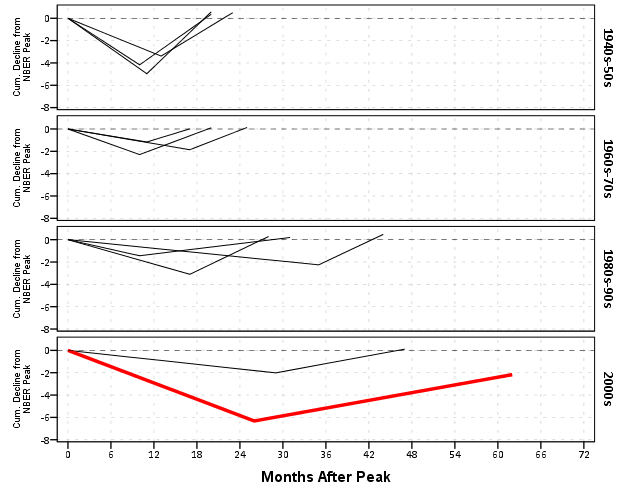Again I lied a bit earlier, you really only needed 14 lines of code to produce the above chart. I actually spent a few saving to a new dataset. I wanted to see if the reduced summary in this dataset was an accurate representation. You can see it is except for years 73 and 80, in which they had slight positive recoveries before the bottoming out, so one bend in the curve doesn’t really cut it in those instances. Again, the chart only takes some slight editing in the GPL to produce. Here I produce a chart where each year has it’s own panel, and the panels are wrapped (instead of placed in new rows). This is useful when you have many panels.

``````compute reduced = 1.
dataset activate NonFarmEmploy.
compute reduced = 0.
/file = 'reduced'.
dataset close reduced.
value labels reduced
0 'Full Series'
1 'Kaisers Reduced Series'.

*for some reason, not letting me format labels for small multiples.
value labels year
'1948' "48"
'1953' "53"
'1957' "57"
'1960' "60"
'1969' "69"
'1973' "73"
'1980' "80"
'1981' "81"
'1990' "90"
'2001' "01"
'2007' "07".

GGRAPH
/GRAPHDATASET NAME="graphdataset" VARIABLES=MAP cdecline year flag_2007 reduced
/GRAPHSPEC SOURCE=INLINE.
BEGIN GPL
SOURCE: s=userSource(id("graphdataset"))
DATA: MAP=col(source(s), name("MAP"))
DATA: cdecline=col(source(s), name("cdecline"))
DATA: year=col(source(s), name("year"), unit.category())
DATA: flag_2007=col(source(s), name("flag_2007"), unit.category())
DATA: reduced=col(source(s), name("reduced"), unit.category())
COORD: rect(dim(1,2), wrap())
SCALE: cat(aesthetic(aesthetic.color), map(("0", color.black), ("1", color.red)))
SCALE: linear(dim(1), min(0), max(72))
SCALE: linear(dim(2), min(-8), max(18))
GUIDE: axis(dim(1), label("Months After Peak"), delta(6))
GUIDE: axis(dim(2), label("Cum. Decline from NBER Peak"), delta(2))
GUIDE: axis(dim(3), opposite())
GUIDE: form.line(position(*,0), size(size."0.5px"), shape(shape.dash), color(color.lightgrey))
GUIDE: legend(aesthetic(aesthetic.color.interior), null())
GUIDE: legend(aesthetic(aesthetic.size), null())
ELEMENT: line(position(MAP*cdecline*year), color(reduced))
END GPL.``````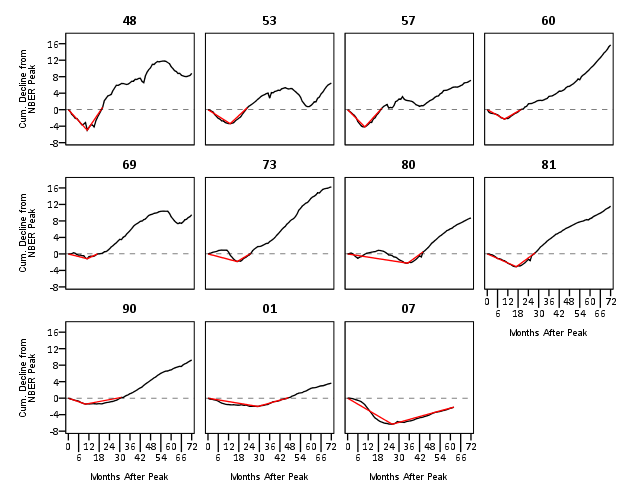SPSS was misbehaving and labelling my years with a comma. To prevent that I made value labels with just the trailing two years. Again I post-hoc edited the size of the Y and X axis labels and manually removed the gridlines. Quite short work. Harder is to add in labels, I don’t bother here, but I would assume to do it nicely (if really needed) I would need to do it manually. I don’t bother here because it isn’t clear to me why I should care

As oppossed to going into a diatribe about the utility of learning a statistical programming language, I will just say that, if you are an analyst that works with data on a regular basis, you are doing yourself a disservice by only sticking to excel. Not only is the tool in large parts limited to the types of graphics and analysis one can conduct, it is very difficult to make tasks routine and reproducible.

Part of my dissapointment is that I highly suspect Kaiser has such programming experience, he just hasn’t taken the time to learn a statistical program thoroughly enough. I wouldn’t care, except that Kaiser is in a position of promoting best practices, and I would consider this to be one of them. I don’t deny that learning such programming languages is not easy, but as an analyst that works with data every day, I can tell you it is certainly worth the effort to learn a statistical programming language well.

# Some random SPSS graph tips: shading areas under curves and using dodging in binned dot plots

This is just a quick post on some random graphing examples you can do with SPSS through inline GPL statements, but are not possible through the GUI dialog. These also take knowing alittle bit about the grammar of graphics, and the nuts and bolts of SPSS’s implementation. First up, shading under a curve.

I assume the motivation for doing this is obvious, but it is alittle advanced GPL to figure out how to accomplish. I swore someone asked how to do this the other day on NABBLE, but I could not find any such questions. Below is an example.

``````*****************************************.
input program.
loop #i = 1 to 2000.
compute X = (#i - 1000)/250.
compute PDF = PDF.NORMAL(X,0,1).
compute CDF = CDF.NORMAL(X,0,1).
end case.
end loop.
end file.
end input program.
dataset name sim.
exe.

formats PDF X (F2.1).

*area under entire curve.
GGRAPH
/GRAPHDATASET NAME="graphdataset" VARIABLES=X PDF MISSING=LISTWISE
REPORTMISSING=NO
/GRAPHSPEC SOURCE=INLINE.
BEGIN GPL
SOURCE: s=userSource(id("graphdataset"))
DATA: X=col(source(s), name("X"))
DATA: PDF=col(source(s), name("PDF"))
GUIDE: axis(dim(1), label("X"))
GUIDE: axis(dim(2), label("Prob. Dens."))
ELEMENT: area(position(X*PDF), missing.wings())
END GPL.

*Mark off different areas.
compute tails = 0.
if CDF <= .025 tails = 1.
if CDF >= .975 tails = 2.
exe.

*Area with particular locations highlighted.
GGRAPH
/GRAPHDATASET NAME="graphdataset" VARIABLES=X PDF tails
/GRAPHSPEC SOURCE=INLINE.
BEGIN GPL
SOURCE: s=userSource(id("graphdataset"))
DATA: X=col(source(s), name("X"))
DATA: PDF=col(source(s), name("PDF"))
DATA: tails=col(source(s), name("tails"), unit.category())
SCALE: cat(aesthetic(aesthetic.color.interior), map(("0",color.white),("1",color.grey),("2",color.grey)))
SCALE: cat(aesthetic(aesthetic.transparency.interior), map(("0",transparency."1"),("1",transparency."0"),("2",transparency."0")))
GUIDE: axis(dim(1), label("X"))
GUIDE: axis(dim(2), label("Prob. Dens."))
GUIDE: legend(aesthetic(aesthetic.color.interior), null())
GUIDE: legend(aesthetic(aesthetic.transparency.interior), null())
ELEMENT: area(position(X*PDF), color.interior(tails), transparency.interior(tails))
END GPL.
*****************************************.``````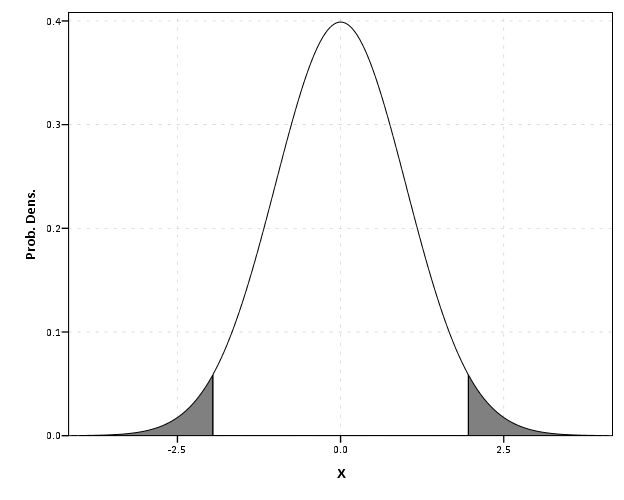The area under the entire curve is pretty simple code, and can be accomplished through the GUI. The shading under different sections though requires a bit more thought. If you want both the upper and lower tails colored of the PDF, you need to specify seperate categories for them, otherwise they will connect at the bottom of the graph. Then you need to map the categories to specific colors, and if you want to be able to see the gridlines behind the central area you need to make the center area transparent. Note I also omit the legend, as I assume it will be obvious what the graph represents given other context or textual summaries.

# Binning scale axis to produce dodging

The second example is based on the fact that for SPSS to utilize the dodge collision modifier, one needs a categorical axis. What if you want the axis to really be scale though? You can make the data categorical but the axis on a continuous scale by specifying a binned scale, but just make the binning small enough to suit your actual data values. This is easy to show with a categorical dot plot. If you can, IMO it is better to use dodging than jittering, and below is a perfect example. If you run the first GGRAPH statement, you will see the points aren’t dodged, although the graph is generated just fine and dandy with no error messages. The second graph bins the X variable (which is on the second dimension) with intervals of width 1. This ends up being exactly the same as the continuous axis, because the values are all positive integers anyway.

``````*****************************************.
set seed = 10.
input program.
loop #i = 1 to 1001.
compute X = TRUNC(RV.UNIFORM(0,101)).
compute cat = TRUNC(RV.UNIFORM(1,4)).
end case.
end loop.
end file.
end input program.
dataset name sim.

GGRAPH
/GRAPHDATASET NAME="graphdataset" VARIABLES=X cat
/GRAPHSPEC SOURCE=INLINE.
BEGIN GPL
SOURCE: s=userSource(id("graphdataset"))
DATA: X=col(source(s), name("X"))
DATA: cat=col(source(s), name("cat"), unit.category())
COORD: rect(dim(1,2))
GUIDE: axis(dim(1), label("cat"))
ELEMENT: point.dodge.symmetric(position(cat*X))
END GPL.

*Now lets try to bin X so the points actually dodge!.
GGRAPH
/GRAPHDATASET NAME="graphdataset" VARIABLES=X cat
/GRAPHSPEC SOURCE=INLINE.
BEGIN GPL
SOURCE: s=userSource(id("graphdataset"))
DATA: X=col(source(s), name("X"))
DATA: cat=col(source(s), name("cat"), unit.category())
COORD: rect(dim(1,2))
GUIDE: axis(dim(1), label("cat"))
ELEMENT: point.dodge.symmetric(position(bin.rect(cat*X, dim(2), binWidth(1))))
END GPL.
****************************************.``````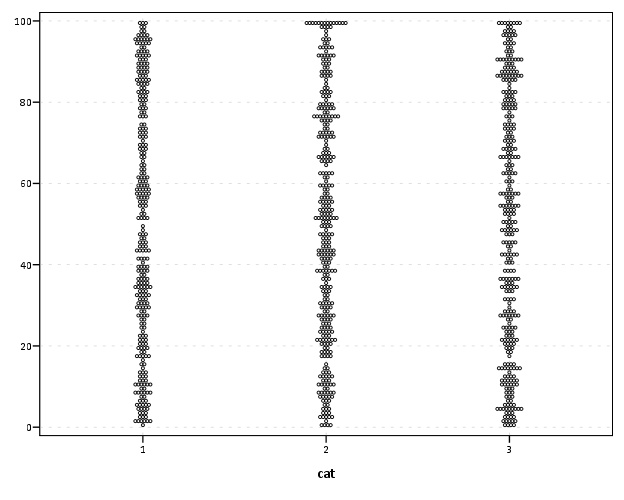Both examples shown here only take slight alterations to code generatable through the GUI, but take a bit more understanding of the grammar to know how to accomplish (or even know they are possible). You unfortunately can’t implement Wilkinson’s (1999) true dot plot technique like this (he doesn’t suggest binning, but by choosing where the dots are placed by KDE estimation). But this should be sufficient for most circumstances.